# KL Divergence in Machine Learning

Nikolaj Buhl
July 26, 2023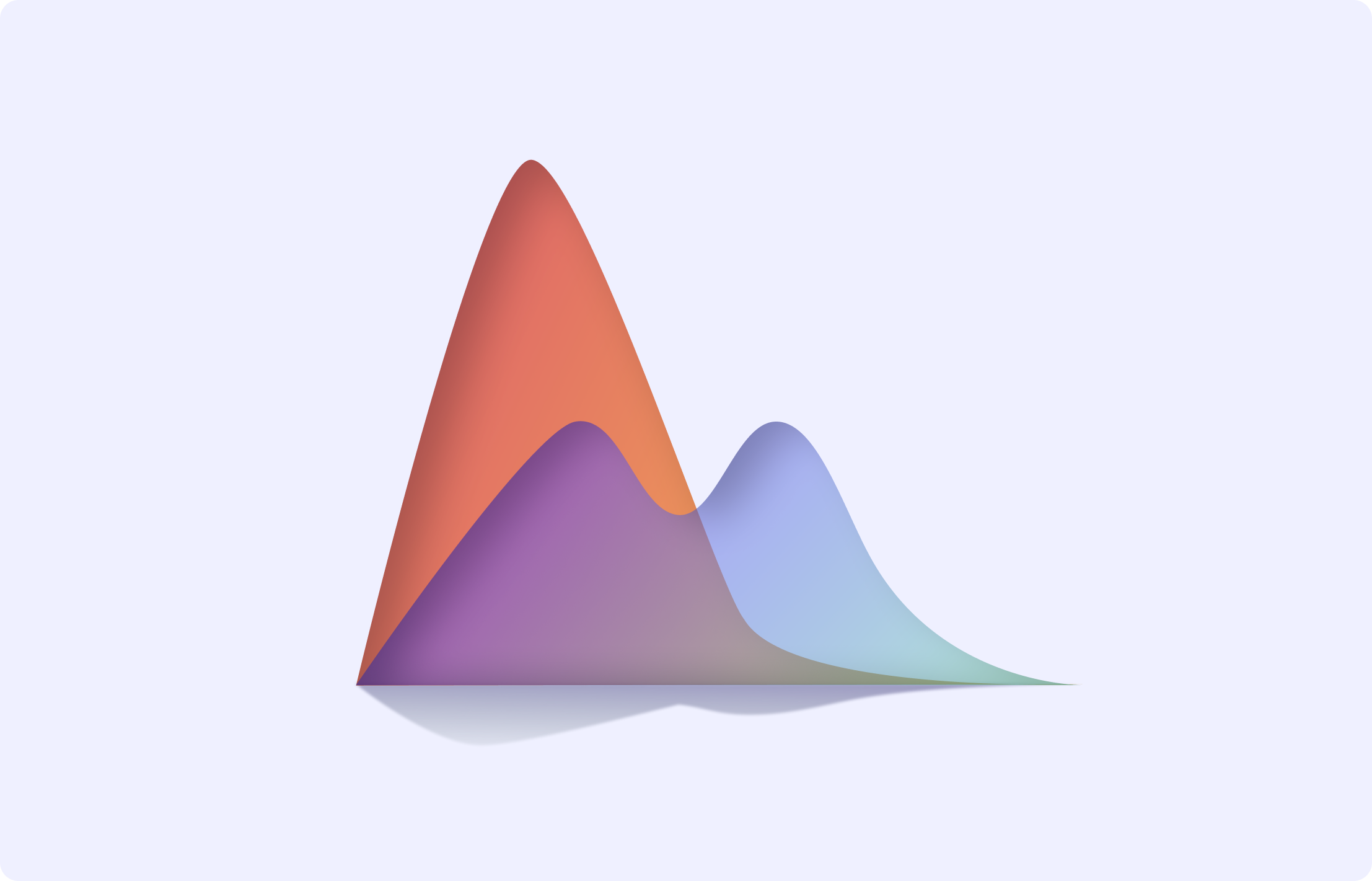Kullback-Leibler (KL) divergence, or relative entropy, is a metric used to compare two data distributions. It is a concept of information theory that contrasts the information contained in two probability distributions. It has various practical use cases in data science, including assessing dataset and model drift, information retrieval for generative models, and reinforcement learning.

## Divergence in Statistics

The divergence between two probability distributions quantifies how much the two differ from each other.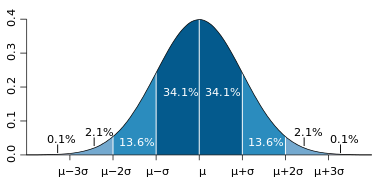Probability Distribution

A probability distribution models the values that a random variable can take. It is modeled using parameters including mean and variance. Changing these parameters gives us different distributions and helps us understand the random numbers' spread in a given latent space.

There are various algorithms for divergence measures, including:

• Jensen-Shannon Divergence
• Hellinger Distance
• Total Variation Divergence
• Kullback-Leibler Divergence

## Mathematics behind Kullback-Leibler KL Divergence

KL divergence is an asymmetric divergence metric. Asymmetric means that given a probability distribution P and a probability distribution Q, the divergence between P and Q will not be the same as Q and P.

KL divergence is defined as the number of bits required to convert one distribution into another. The lower bound value is zero and is achieved when the distributions under observation are identical.

It is often denoted with the following notation:

• D KL(P||Q),

and the formula for the metric is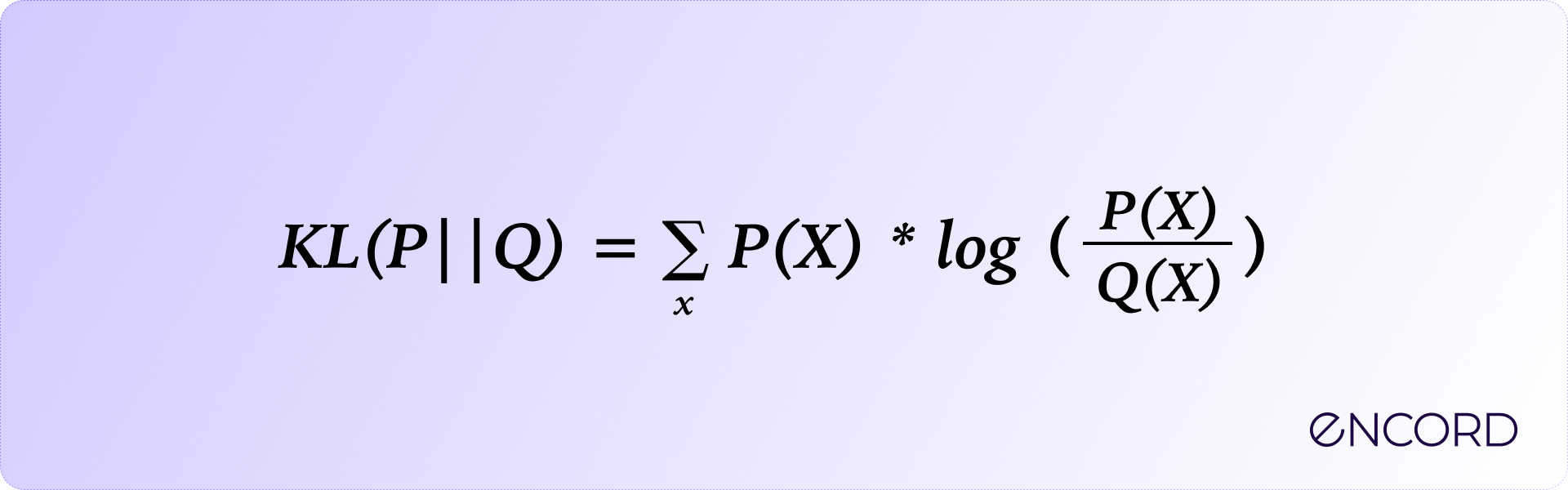In the formula above:

• X is a possible event in the probability space.
• P(x) and Q(x) are the probabilities of x in the distribution of P and Q, respectively.
• The ratio P(x)/Q(x) represents the relative likelihood of event "x" according to P compared to Q.

The formula shows that KL divergence closely resembles the cross-entropy loss function used in deep learning. This is because both KL divergence and cross-entropy are quantification of the differences between different data distributions and are often interchangeable. Let's expand the equation to understand the relationship further.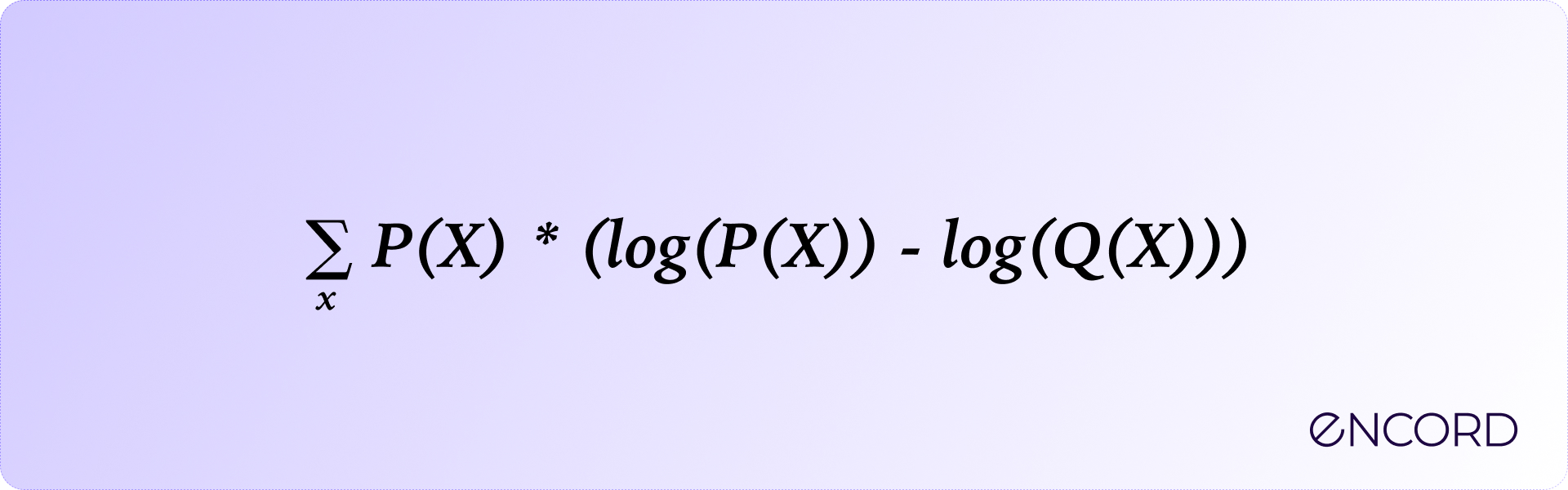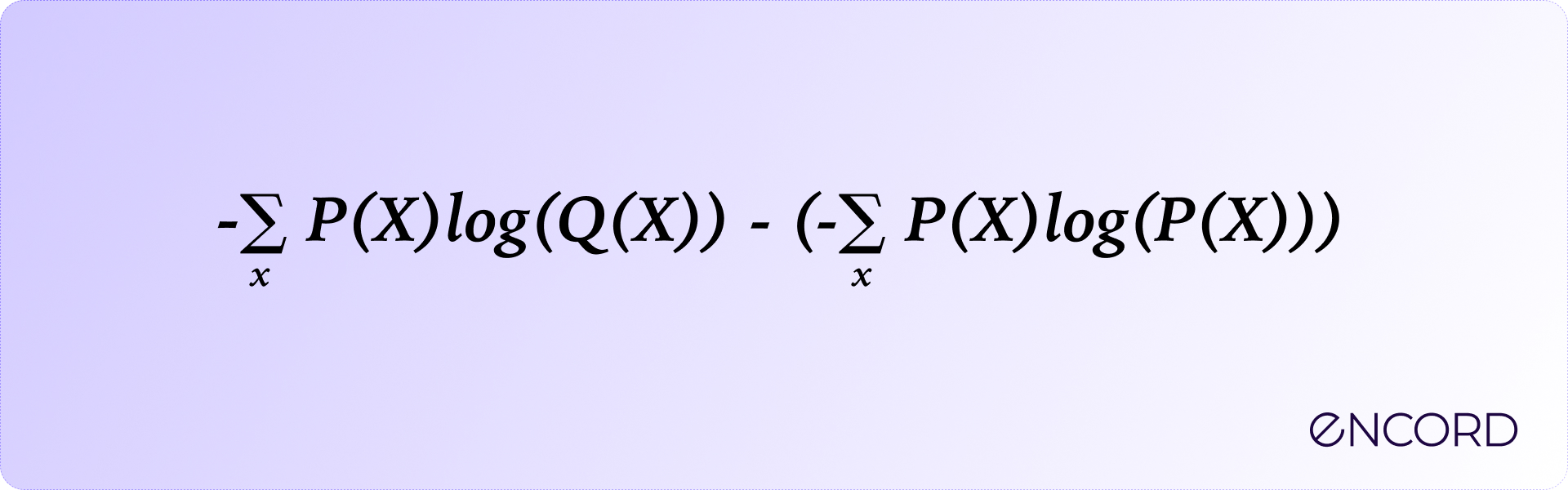The term to the right is the entropy H(X) of the probability distribution P(X) while that to the left is the cross-entropy between P(X) and P(Q).

## Applications of KL Divergence in Data Science

### Monitoring Data Drift

One of the most common use cases of KL divergence in machine learning is to detect drift in datasets. Data is constantly changing, and a metric is required to assess the significance of the changes.

Constantly monitoring data allows machine learning teams to decide whether the model re-training is required. It also provides insights regarding the variable nature of the data under consideration and helps draw statistical analysis.

Scale your annotation workflows and power your model performance with data-driven insights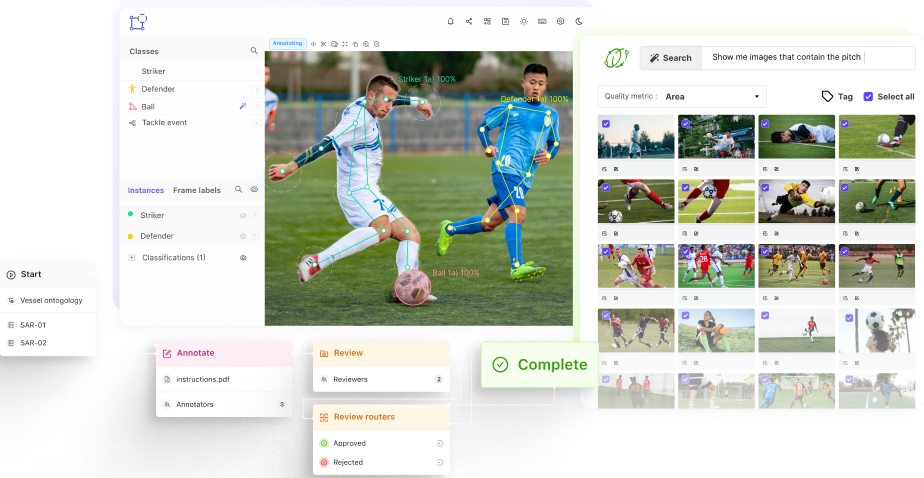KL divergence is applied to data in discrete form by forming data bins. The data points are binned according to the features to form discrete distributions, i.e., each feature is independently processed for divergence calculation. The divergence scores for each bin are summed up to get a final picture.

### Loss Function for Neural Networks

While regression problems use the mean-squared error (MSE) loss function for the maximum likelihood estimation, a classification problem works with probabilistic distributions. A classification neural network is coupled with the KL divergence loss function to compare the model outputs to the true labels

For example, a binary cat classifier predicts the probabilities for an image as p(cat) = 0.8 and p(not cat) = 0.2. If the ground truth of the image is that it is a cat, then the true distribution becomes p(cat) = 1 and p(not cat) = 0. We now have two different distributions modeling the same variable. The neural network aims to bring these predicted distributions as close to the ground truth as possible.

Taking values from our example above as P(X) = {1,0} and Q(X) = {0.8, 0.2}, the divergence would be: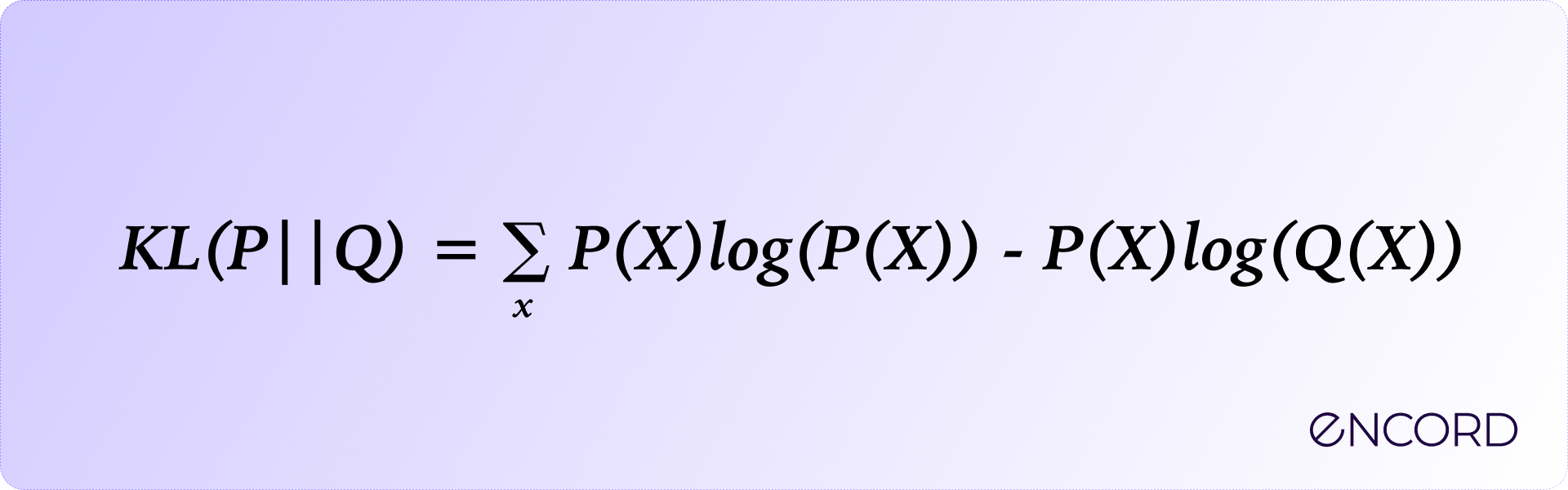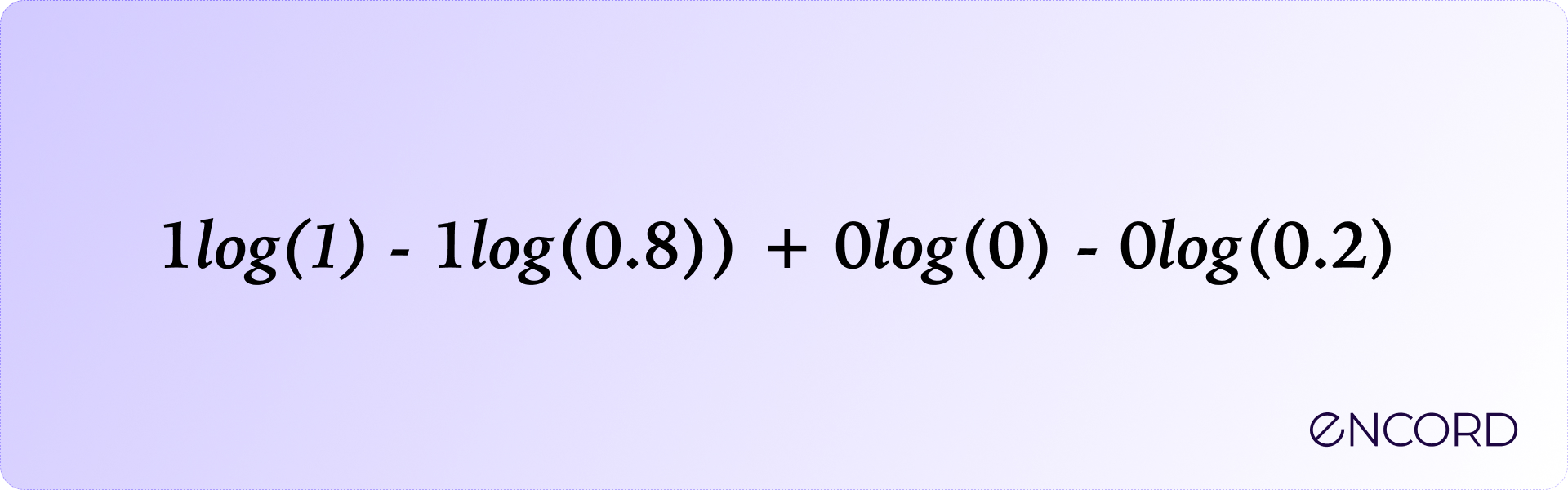Most of the terms above will be zeroed out and the final result comes out to be:When using KL divergence as the loss, the network optimizes to bring the divergence value down to zero.

### Variational Auto-Encoder Optimization

An auto-encoder is a neural network architecture that encodes an input image onto an embedding layer. Variational auto-encoders (VAEs) are a specialized form of traditional architecture that project the input data onto a probability distribution (usually a Gaussian distribution).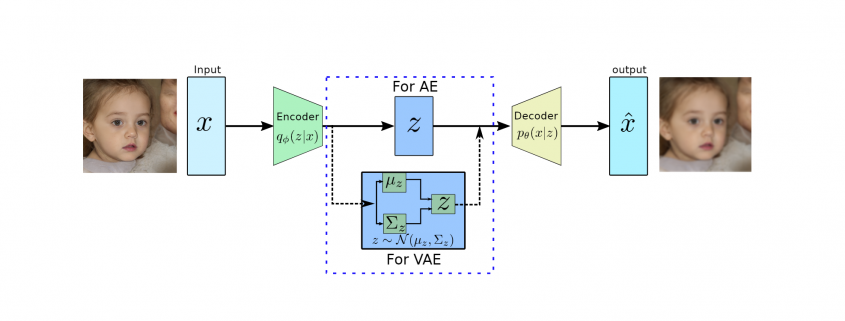Variational Autoencoders

The VAE architecture involves two loss functions, a mean-squared error (MSE) to calculate the loss between the output image and the ground truth and the KL divergence to calculate the statistical distance between the true distribution and the approximating distribution in the middle.Interested in encodings? Here’s our Full Guide to Embeddings.

The Generative Adversarial Networks (GANs) consist of two networks training to outdo each other (adversaries). One of the networks trains to output synthetic (fake) data indistinguishable from the actual input, while the other learns to detect synthetic images from actual.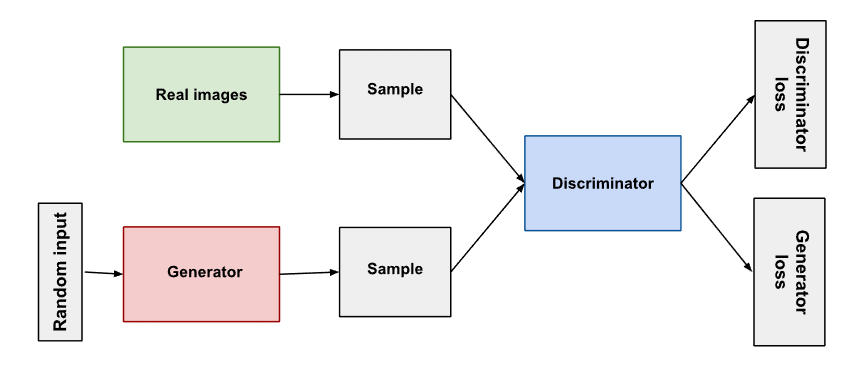The datasets involved in GANs (fake and real) are two distributions the network tries to compare. The comparison uses KL divergence to create a comparable metric to evaluate whether the model is learning. The discriminator tries to maximize the divergence metric, while the generator tries to minimize it, forming an overall min-max loss configuration.

Moreover, it is essential to mention that KL divergence does have certain drawbacks, such as its asymmetric behavior and unstable training dynamics, that make the Jensen-Shannon Divergence a better fit.

## Limitations of KL Divergence

As previously established, KL divergence is an asymmetric metric. This means that it can not be used as strictly a distance measure since the distance between two entities remains the same from either perspective.

Moreover, if the data samples are pulled from distributions that use different parameters (mean and variance), KL divergence will not yield reliable results. In this case, one of the distributions needs to be adjusted to match the other.

## KL Divergence: Key Takeaways

• Divergence is a measure that provides the statistical distance between two distributions.
• KL divergence is an asymmetric divergence metric defined as the number of bits required to convert one distribution into another.
• A zero KL divergence score means that the two distributions are exactly the same. A higher score defines how different the two distributions are.
• KL divergence is used in artificial intelligence as a loss function to compare the predicted data with true values.
• Some other AI applications include generative adversarial networks (GANs) and data model drifting.Written by Nikolaj Buhl
Nikolaj is a Product Manager at Encord and a computer vision enthusiast. At Encord he oversees the development of Encord Active. Nikolaj holds a M.Sc. in Management from London Business School and Copenhagen Business School. In a previous life, he lived in China working at the Danish Embas... see more#### Discuss this blog on Slack

Join the Encord Developers community to discuss the latest in computer vision, machine learning, and data-centric AI

Join the community
###### Subscribe